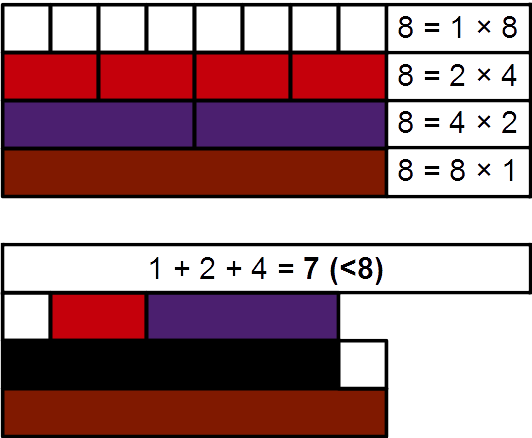# What is a Deficient Number?

Instructor: Danielle Wilson

Danielle is a certified elementary, middle school math, and special education teacher. She has a master's degree in elementary education and special education.

A deficit is when you don't have enough of something to get what you want or for it to be complete. Did you know that numbers can be deficient? Read along in this lesson to find out about the numbers that just aren't quite complete.

## Oh No! Not Deficient!

It's important to eat a healthy diet of fruits, vegetables, protein, and dairy products to make sure you get all the nutrients and vitamins your body needs to grow strong. If you don't get enough of these vitamins from your diet, you may become deficient. When your body becomes deficient, it lacks the important nutrients you need to be healthy and strong. Did you know numbers can also be deficient? Let's learn what it means when a number is deficient.

## Deficient Numbers

Deficient numbers are numbers that don't have much value when broken down into a sum of its proper factors. For example, the proper factors of 8 are 1, 2, and 4. This is because a proper factor is any factor of a number except the number itself. So, 8 is just a factor of itself, it is not a proper factor of 8. Now, if I add the proper factors of 8 together, I will get another number. Right? If this new number is less than 8, then 8 is a deficient number. So let's add 1 + 2 + 4 = 7. The number 7 is less than 8. So, this means that 8 is a deficient number! Look at the image below which uses Cuisenaire rods to demonstrate just how the number 8 is deficient.## Some Fun Examples

Let's figure out if the number 21 is a deficient number. Are you ready?!

1, 3, and 7

### Step 2: Add the proper factors together to see if the sum is less than 21.

1 + 3 + 7 = 11

11 is less than 21. So, this means that 21 is a deficient number! Wow!

Now let's figure out if the number 36 is deficient.

### Step 1: List the proper factors of 36.

1, 2, 3, 4, 6, 9, 12, and 18

### Step 2: Add the proper factors together to see if the sum is less than 36.

1 + 2 + 3 + 4 + 6 + 9 + 12 + 18 = 45

Now 45 is larger than 36. So, 36 is not a deficient number!

To unlock this lesson you must be a Study.com Member.

### Register to view this lesson

Are you a student or a teacher?

#### See for yourself why 30 million people use Study.com

##### Become a Study.com member and start learning now.
Back
What teachers are saying about Study.com

### Earning College Credit

Did you know… We have over 200 college courses that prepare you to earn credit by exam that is accepted by over 1,500 colleges and universities. You can test out of the first two years of college and save thousands off your degree. Anyone can earn credit-by-exam regardless of age or education level.